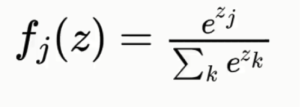# Decoding Softmax Activation Function for Neural Network with Examples in Numpy, Keras, TensorFlow and PyTorch## Introduction

Activation functions are an integral part of the neural network that determines how the model behaves. There are many activation functions that are available as choices which often leads to confusion for beginners. In this article, we will discuss and explain to you the characteristics of the Softmax activation function along with examples in Numpy, Keras, TensorFlow, and PyTorch.

We will keep the explanation at intuition level only for beginners. So let us get started.

## What is Activation Function in Neural Network?In neural networks, the activation function is used to transforms the incoming signals of an artificial neuron to produce an output signal. Activation functions are essential to add nonlinearity to the network to produce models that can understand complex nonlinear relationships of real-world data.

## Softmax Activation Function

Softmax activation function converts the input signals of an artificial neuron into a probability distribution.

It is usually used in the last layer of the neural network for multiclass classifiers where we have to produce probability distribution for classes as output.

As you can see in the below illustration, the incoming signal from the previous hidden layer is passed through the softmax activation function and it produces probability distribution for the three classes. The class with the highest probability is chosen as the predicted class.## Softmax Activation Function Formula

Mathematically, it is a generalized version of the sigmoid function (another activation function) and its formula is as below –Here z is the vector which is the input to the neuron and k is the number of classes in the multiclass classification problem.

## Softmax Function in Numpy

You can use Numpy library to create calculate Softmax function as below –

### Example

```import numpy as np

input = [3,4,1]

output=np.exp(input - np.max(input))/np.exp(input - np.max(input)).sum()
output```

Output:

`array([0.25949646, 0.70538451, 0.03511903])`

## Softmax Function in TensorFlow

In TensorFlow, you can apply Softmax function by using tf.nn.softmax() function.

### Example

```import tensorflow as tf

input = tf.constant([[3,4,1]], dtype = tf.float32)

output = tf.nn.softmax(a)
output.numpy()```

Output:

`array([[0.25949648, 0.7053845 , 0.03511902]], dtype=float32)`

You can also create your own custom formula to calculate Softmax function as shown in the below example-

### Example

```import tensorflow as tf
input = tf.constant([[3,4,1]], dtype = tf.float32)

output = tf.exp(inputs) / tf.reduce_sum(tf.exp(inputs), axis=1)
output.numpy()```

Output:

`array([[0.25949645, 0.7053845 , 0.03511903]], dtype=float32)`

## Softmax Function in Keras

In TF Keras, you can apply Softmax function by using tf.keras.activations.softmax() function.

### Example

```import tensorflow as tf

inputs = tf.constant([[3,4,1]], dtype = tf.float32)

outputs = tf.keras.activations.softmax(inputs)
outputs.numpy()```

Output:

`array([[0.25949648, 0.7053845 , 0.03511902]], dtype=float32)`

While working with Keras dense layers it is quite easy to add the softmax activation function as shown below –

``layer = tf.keras.layers.Dense(32, activation=tf.keras.activations.softmax)``

## Softmax Function in PyTorch

In PyTorch, the Softmax function can be implemented by using nn.Softmax() function as shown in the example below –

```from torch import nn

m = nn.Softmax(dim=1)
input = torch.tensor([[3.0, 4.0, 1.0]],dtype=torch.float)
#input = torch.randn(1, 3)
output = m(input)
output```

Output:

`tensor([[0.2595, 0.7054, 0.0351]])`

## Conclusion

In this article, we explained what is Softmax activation function is and what are its characteristics. We also covered practical examples of various functions of Numpy, Keras, TensorFlow, and PyTorch for Softmax.

References-